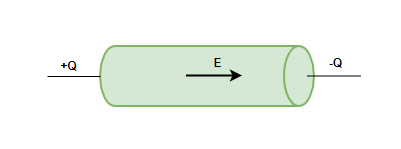GFG App
Open AppBrowser
Continue

# Electric Current in Conductors

Charges are present in every living and non-living being on earth. In earlier centuries, charges were considered to be rest. But in the 19th century, a new phenomenon was observed about moving charges. It was observed that moving charges constitute a current. These currents occur in many situations in nature, for example – a lightning strike is actually a current that flows from clouds up to the ground. In everyday lives, people encounter current in many electric devices.

### Electric Current

The devices in our everyday use carry electric currents inside of them. Torches, bulbs, fans, heaters, etc are some examples of electric devices which have electric currents flowing into them. Let’s imagine charges are flowing in the horizontal direction, in this flow assume a surface with a small area held normal to the direction of flow of charges. Assume both positive and negative charges are flowing across this area.

In a given time interval “t”, the charge passing through the surface can be divided into two parts – positive charges and negative charges. Let’s say q+ is the net positive charge passing through the surface in the given time interval, similarly q is the net negative charge passing through the surface in the given time interval. In this case, the net charge passing through this surface in this interval will be,

qnet =  q+ – q

Electric current in defined as the rate of flow of charge in a conductor.

In this case, the average current flowing through the given surface in time “t” is given by,Here, I represents the average current flowing through the surface.

The unit for current is Ampere (A), Ampere stands for Column/sec.

In case when the rate of flow of charge is varying, the current flowing is given by,⇒### Electric Currents in Conductors

An electric charge experiences force if an electric field is applied, due to the force it starts moving and the movement of these charges constitutes the electric current. In a solid conductor, atoms are tightly bound with each other and approximately all the electrons are bound to the atoms. There are some electrons that are free from all the atoms are able to move freely throughout the material. When no electric field is applied, these electrons perform motion in random directions. At a given time, there is no preferential direction for the velocities of the electrons. This means, on average the number of electrons travelling in any direction will be equal to the number of electrons travelling in the opposite direction. So, there will be no net electric current.Let’s see how these electrons behave when an electric field is applied to the conductor. Imagine a conductor given in the figure above, suppose one is a positively charges cylindrical disc and a negatively charged cylindrical disc. These discs are kept at the ends of a cylindrical conductor. An electric field will be created inside the conductor, this field will exert the force of charges and they will move. The movement of charges causes the electric current

### Sample Problems

Question 1: Assume a horizontal plate and positive charge of 50C flows in 5 seconds through that plate. Find the magnitude of the electric current passing through that plate.

It is known that electric current is the rate of charge passing through the conductor.

Net charge passing through the conductor qnet = 50C

Time taken, t = 5⇒⇒ I = 10 A

Question 2: Assume a horizontal plate and positive charge of 20C flows in 2.5 seconds through that plate. Find the magnitude of the electric current passing through that plate.

It is known that electric current is the rate of charge passing through the conductor.

Net charge passing through the conductor qnet = 20C

Time taken, t = 2.5⇒⇒ I = 8 A

Question 3: The charge present inside the conductor at a given time is given by the function q(t) below.

q(t) = sin(t)

Find the current flowing through the conductor at time t.

In this case, the rate of charge through the conductor is changing. So, average current cannot be calculated, for such case instantaneous current is calculated.⇒⇒ I = cos(t) A

Question 4: The charge present inside the conductor at a given time is given by the function q(t) below.

q(t) = t2 + 2t

Find the current flowing through the conductor at time t = 2.

In this case, the rate of charge through the conductor is changing. So, average current cannot be calculated, for such case instantaneous current is calculated.⇒⇒⇒ I = 2t + 2

at t = 2.

I = 2(2) + 2

⇒ I = 4 + 2

⇒ I = 6 A

Question 5: The charge present inside the conductor at a given time is given by the function q(t) below.

q(t) = t + 2t3 + et

Find the current flowing through the conductor at time t = 1.

In this case, the rate of charge through the conductor is changing. So, average current cannot be calculated, for such case instantaneous current is calculated.⇒⇒ )⇒ I = 1 + 6t2 + et

at t = 1.

I = 1 + 6t2 + et

⇒ I = 1 + 6(1) + e

⇒ I  = 7 + e

⇒ I = 9.7A

My Personal Notes arrow_drop_up